# NCERT Solutions for Class 9 Maths Chapter 8 - Quadrilaterals

Share

NCERT Solutions For Class 9 Maths Chapter 8 – Quadrilaterals made available by Goprep provide students with the opportunity to prepare the Chapter thoroughly. These Solutions for Chapter 8 have been developed in sync with the latest syllabus of the Class 9 Maths suggested by the CBSE. These Solutions are highly valuable, and students can use them to clear their doubts and develop their question-solving ability.

Chapter 8 of the Class 9 Maths textbook deals with the introduction of the Quadrilaterals, Angle Sum Property Of A Quadrilateral, Types Of Quadrilaterals, Properties Of A Parallelogram and The MidPoint Theorem among others. Given in a simple and structured manner, our NCERT Solutions can help you a great deal in developing a thorough understanding of each topic. So, if you want to prepare well for the exam, then referring to these Solutions can surely help facilitate your exam preparation in the best direction.

## NCERT Solutions for Class 9 Maths Chapter 8 - Quadrilaterals

Exercise 8.1
• Exercise 8.1
• Exercise 8.2
Class 9th|NCERT - MathematicsChapter 8 - Quadrilaterals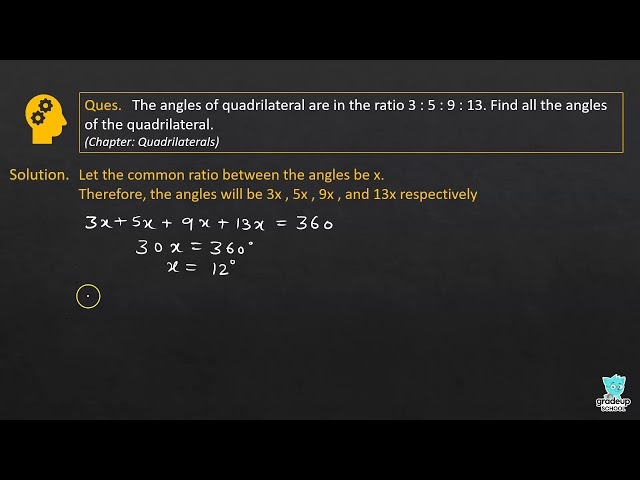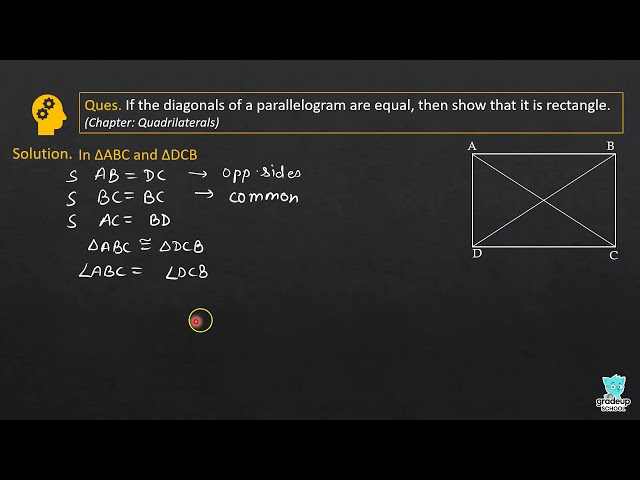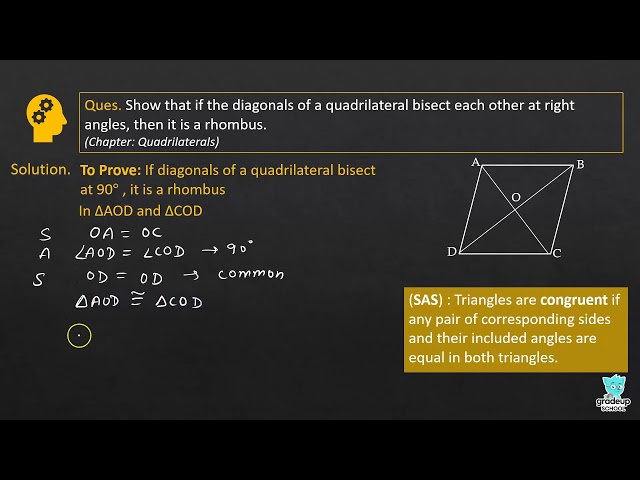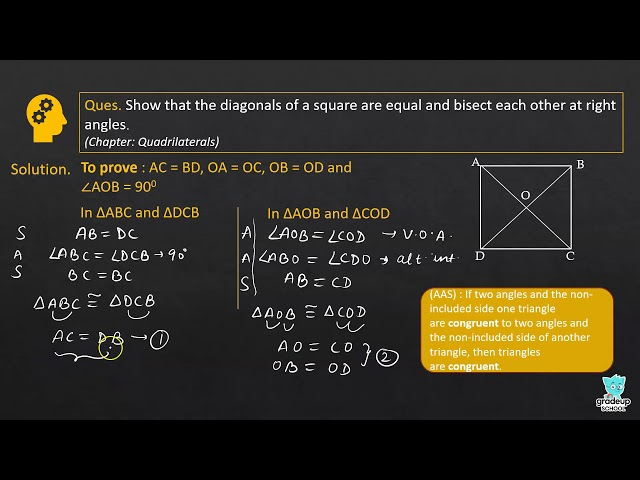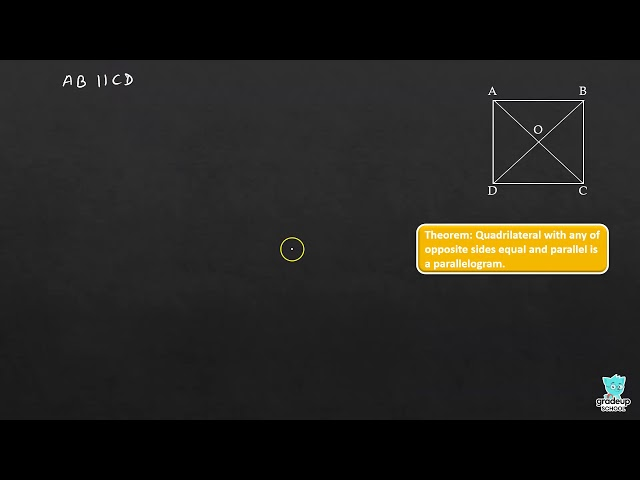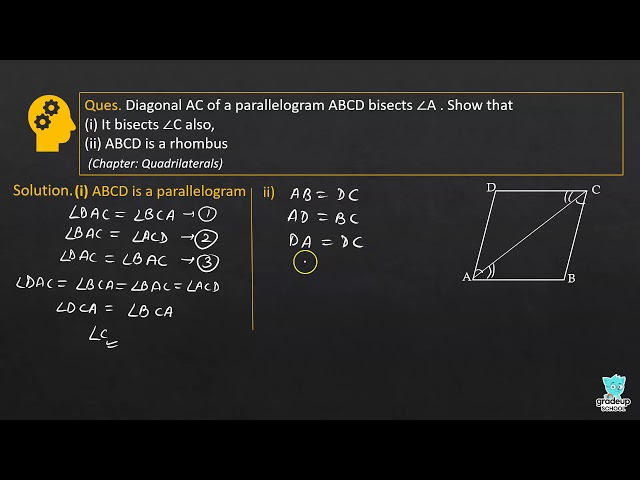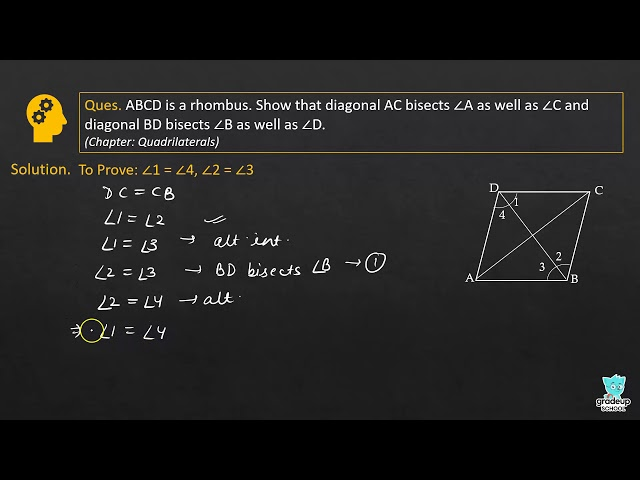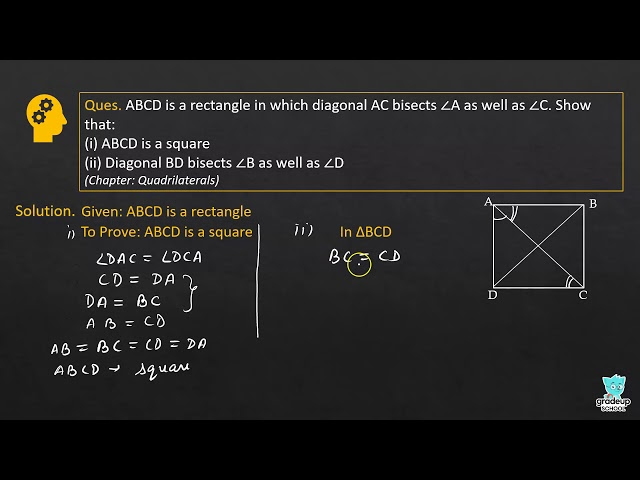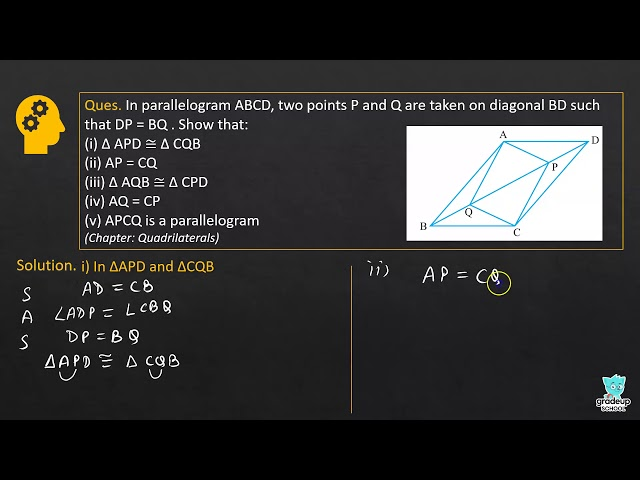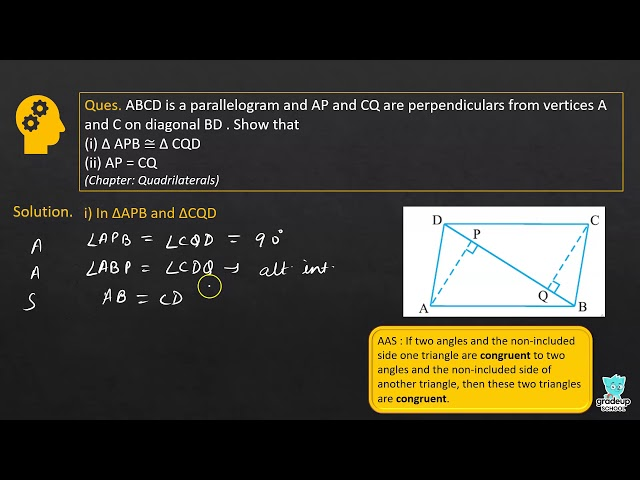See More (2)
NCERT - MathematicsClass 9th , NCERT
 Chapter 1 - Number System Chapter 2 - Polynomials Chapter 3 - Coordinate Geometry Chapter 4 - Linear Equations in two Variables Chapter 5 - Introduction to Euclid's Geometry Chapter 6 - Lines and Angles Chapter 7 - Triangles Chapter 8 - Quadrilaterals Chapter 9 - Areas of Parallelograms and Triangles Chapter 10 - Circles Chapter 11 - Constructions Chapter 12 - Heron's Formula Chapter 13 - Surface Areas and Volumes Chapter 14 - Statistics Chapter 15 - Probability